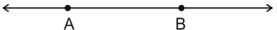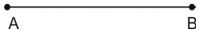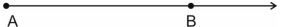# Lines and Angles class 9 Notes Mathematics## myCBSEguide App

CBSE, NCERT, JEE Main, NEET-UG, NDA, Exam Papers, Question Bank, NCERT Solutions, Exemplars, Revision Notes, Free Videos, MCQ Tests & more.

CBSE class 9 Mathematics Chapter 6 Lines and Angles notes in PDF are available for free download in myCBSEguide mobile app. The best app for CBSE students now provides Lines and Angles class 9 Notes latest chapter wise notes for quick preparation of CBSE exams and school based annual examinations. Class 9 Mathematics notes on Chapter 6 Lines and Angles are also available for download in CBSE Guide website.

## CBSE Guide Lines and Angles class 9 Notes

CBSE guide notes are the comprehensive notes which covers the latest syllabus of CBSE and NCERT. It includes all the topics given in NCERT class 9 Mathematics text book. Users can download CBSE guide quick revision notes from myCBSEguide mobile app and my CBSE guide website.

## 10 Mathematics notes Chapter 6 Lines and Angles

Download CBSE class 9th revision notes for Chapter 6 Lines and Angles in PDF format for free. Download revision notes for Lines and Angles class 9 Notes and score high in exams. These are the Lines and Angles class 9 Notes prepared by team of expert teachers. The revision notes help you revise the whole chapter in minutes. Revising notes in exam days is on of the best tips recommended by teachers during exam days.

CBSE Class 09 Mathematics
Revision Notes
CHAPTER – 6
LINES AND ANGLES

1. Basic Terms and Definitions
2. Intersecting Lines and Non-intersecting Lines
3. Pairs of Angles
4. Parallel Lines and a Transversal
5. Lines Parallel to the same Line
6. Angle Sum Property of a Triangle

(1) Point – We often represent a point by a fine dot made with a fine sharpened pencil on a piece of paper.

(2) Line – A line is completely known if we are given any two distinct points. Line AB is represented by as  . A line or a straight line extends indefinitely in both the directions.(3) Line segment – A part (or portion) of a line with two end points is called a line segment.(4) Ray – A part of line with one end point is called a ray.It usually denotes the direction of line(5) Collinear points – If three or more points lie on the same line, they are called collinear points, otherwise they are called non-collinear points.

(6) Angle – An angle is the union of two non-collinear rays with a common initial point.

Types of Angles –

(1) Acute angle – An acute angle measure between  and

(2) Right angle – A right angle is exactly equal to

(3) Obtuse angle – An angle greater than  but less than

(4) Straight angle – A straight angle is equal to

(5) Reflex angle – An angle which is greater than  but less than  is called a reflex angle.

(6) Complementary angles – Two angles whose sum is  are called complementary angles. Let one angle be x, then its complementary angle be

(7) Supplementary angle – Two angles whose sum is  are called supplementary angles. Let one angle be x, then its supplementary angle be

(8) Adjacent angles -Two angles are Adjacent when they have a common side and a common vertex (corner point) and don’t overlap..

(9) Linear pair – A linear pair of angles is formed when two lines intersect. Two angles are said to be linear if they are adjacent angles formed by two intersecting lines. The measure of a straight angle is 180 degrees, so a linear pair of angles must add up to 180 degrees

(10) Vertically opposite angles – Vertically opposite angles are formed when two lines intersect each other at a point. Vertically opposite angles are always equal.

TRANSVERSAL – A line which intersects two or more given lines at distinct points, is called a transversal of the given line.

(a) Corresponding angles

(b) Alternate interior angles

(c) Alternate exterior angles

(d) Interior angles on the same side of the transversal.

• If a transversal intersects two parallel lines, then

(i) each pair of corresponding angles is equal.

(ii) each pair of alternate interior angles is equal.

(iii) each pair of interior angle on the same side of the transversal is supplementary.

• If a transversal interacts two lines such that, either

(i) any one pair of corresponding angles is equal, or

(ii) any one pair of alternate interior angles is equal or

(iii) any one pair of interior angles on the same side of the transversal is supplementary ,then the lines are parallel.

• Lines which are parallel to a given line are parallel to each other.
• The sum of the three angles of a triangle is
• The sum of all angles round a point is equal to
• If a side of a triangle is produced, the exterior angle so formed is equal to the sum of the two interior opposite angles.
• If two parallel lines are intersected by a transversal, the bisectors of any pair of alternate interior angles are parallel and vice-versa.
• If two parallel lines are intersected by a transversal, then bisectors of any two corresponding angles are parallel and vice-versa.
• If a line is perpendicular to one of the given parallel lines, then it is also perpendicular to the other line.

## Lines and Angles class 9 Notes

• CBSE Revision notes for Class 9 Mathematics PDF
• CBSE Revision notes Class 9 Mathematics – CBSE
• CBSE Revisions notes and Key Points Class 9 Mathematics
• Summary of the NCERT books all chapters in Mathematics class 9
• Short notes for CBSE class 9th Mathematics
• Key notes and chapter summary of Mathematics class 9
• Quick revision notes for CBSE board exams

## CBSE Class-9 Revision Notes and Key Points

Lines and Angles class 9 Notes. CBSE quick revision note for Class-9 Mathematics, Chemistry, Maths, Biology and other subject are very helpful to revise the whole syllabus during exam days. The revision notes covers all important formulas and concepts given in the chapter. Even if you wish to have an overview of a chapter, quick revision notes are here to do if for you. These notes will certainly save your time during stressful exam days.

To download Lines and Angles class 9 Notes, sample paper for class 9 Mathematics, Social Science, Science, English Communicative; do check myCBSEguide app or website. myCBSEguide provides sample papers with solution, test papers for chapter-wise practice, NCERT solutions, NCERT Exemplar solutions, quick revision notes for ready reference, CBSE guess papers and CBSE important question papers. Sample Paper all are made available through the best app for CBSE students and myCBSEguide website.